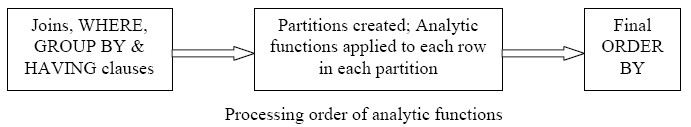# Function - Maximum (Max)

A page about the max aggregate function

Discover More(Function|Operator) - Associative Property

An operator or functionop is associative if the following holds: The importance of this to parallel evaluation can be seen if we expand this to four terms: So we can evaluate (a op b) in parallel...Data Processing - Selection

selection is a data processing operation. finding: the min, the max the median or even any kth element in sub-linear timeFunction - (Aggregate | Aggregation)

Aggregate functions return a single value calculated or selected from values that are in a aggregation relationship (ie a set) This values are also known as summary because they try to summarize...How to use the Aggregate / Window Functions (sum, avg, ) in Sqlite ?

... The aggregate / window function in Sqlite. Sqlite supports the following aggregate /window function : SUM (total) AVG Max Min Rank row_number more see the Specification...
Javascript - Maximum

in javascript Numerical order comparison From d3-array d3.max is a the natural order comparison. ie the number 20 is bigger than...SQL Function - Window Aggregate (Analytics function)

Windowing functions (known also as analytics) allow to compute: cumulative, moving, and aggregates. They are distinguished from ordinary SQL functions by the presence of an OVER clause. With...Statistics - (Data|Data Set) (Summary|Description) - Descriptive Statistics

Summary are a single value summarizing a array of data. They are: selected or calculated through reduction operations. They are an important element of descriptive analysis One of the most important...